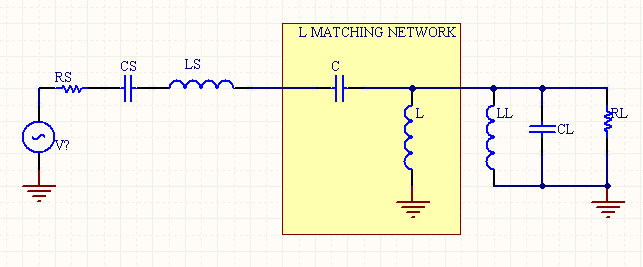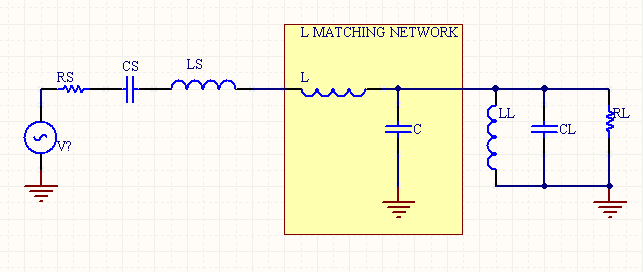# L Matching Network Calculator

The following is a design tool which calculates the values for an L matching network.

The Q (bandwidth) of a filter is fixed for an L matching network, because it is set by the ratio of the source and load resistance which are typically fixed values. For a variable Q use a Pi or T matching network.

This calculator allows for complex source and load impedances, or purely resistive impedances. You can enter in capacitive or inductive components to the load and source reactance, but not both. For example, if the load is capacitive, then enter in a value for the load capacitor, but not the inductor value.

 RS (Source Resistance) (Ohms) CS (Source Capacitance) (pF) (Leave Blank if unused.) LS (Source Inductance) (uH) (Leave Blank if unused.) RL (Load Resistance) (Ohms) CL (Load Capacitance) (pF) (Leave Blank if unused.) LL (Load Inductance) (uH) (Leave Blank if unused.) Frequency (MHz) Block DC Yes, NoFigure 1. L Matching circuit that blocks DCFigure 2. L Matching circuit that passes DC.

[Plant Database], [Soil Moisture Sensor] [Water Level Sensor] [Soil Moisture Meter]